Do you like the article?
Share it with others -

Use new possibilities of MetaTrader 5

# Triangular arbitrage

30 November 2017, 13:26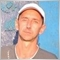28
22 126

### General idea

Topics devoted to the triangular arbitrage appear on forums with unfailing regularity. So, what is it exactly?

The "arbitrage" implies some neutrality towards the market. "Triangular" means that the portfolio consists of three instruments.

Let's take the most popular example: the "EUR — GBP — USD" triangle. In terms of currency pairs, it may be described as follows: EURUSD + GBPUSD + EURGBP. The reuiqred neutrality consists in an attempt to buy and sell the same instruments simultaneously while earning profit.

This looks as follows. Any pair from this example is represented through the other two:

EURUSD=GBPUSD*EURGBP,

or GBPUSD=EURUSD/EURGBP,

or EURGBP=EURUSD/GBPUSD.

All these variants are identical, and the choice of any of them is discussed in more detail below. In the meantime, let's consider the first option.

First, we need to look at bid and ask prices. The procedure is as follows:

1. Buy EURUSD, i.e. use the ask price. This means, we add EUR to our balance while getting rid of USD.
2. Let's evaluate EURUSD through other two pairs.
3. GBPUSD: there is no EUR here. Instead, there is USD we need to sell. In order to sell USD in GBPUSD, we need to buy the pair. This means, we use ask. When buying, we add GBP to our balance, while getting rid of USD.
4. EURGBP: we need to buy EUR and sell GBP that we do not need. Buy EURGBP, use ask. We add EUR to our balance, while getting rid of GBP.

In total we have: (ask) EURUSD = (ask) GBPUSD * (ask) EURGBP. We have obtained the necessary equality. To use it for making profit, we should buy one side and sell the other. There are two possible options here:

1. Buy EURUSD cheaper than we can sell it, but shown in a different way: (ask) EURUSD < (bid) GBPUSD * (bid) EURGBP
2. Sell EURUSD at a higher price than we can buy, but shown in a different way: (bid) EURUSD > (ask) GBPUSD * (ask) EURGBP

Now, all we have to do is detect such a case and make profit on it.

Note that the triangle can be made in another way by moving all three pairs in one direction and comparing with 1. All variants are identical, but I believe, the one described above is easier to perceive and explain.

By tracking the situation, we can search for a moment for simultaneous buying and selling. In this case, the profit will be instant, but such moments are rare.
More common are the cases when we are able to buy one side cheaper but are not able to sell it with a profit right now. Then we wait for this imbalance to disappear. Being in a trade is safe for us, since our position is almost zero, meaning we are out of the market. Although, note the word "almost" here. For a perfect leveling of trade volumes, we need a precision that is not available to us. Trade volumes are most often rounded to two decimal places which is too rough for our strategy.

Now that we have considered the theory, it is time to write an EA. The EA is developed in a procedural style, so it is understandable for both novice programmers and those who for some reason do not like OOP.

### Brief EA description

First, we create all possible triangles, place them correctly and get all the necessary data for each currency pair.

All this information is stored in the MxThree array of structures. Each triangle has the status field. Its initial value is 0. If the triangle needs to be opened, the status is set to 1. After confirming that the triangle opened completely, its status changes to 2. If the triangle opens partially or it is time to close it, the status changes to 3. Once the triangle is successfully closed, the status returns to 0.

Opening and closing triangles are saved to a log file allowing us to check the correctness of actions and restore history. The log file name is Three Point Arbitrage Control YYYY.DD.MM.csv.

To perform a test, upload all necessary currency pairs to the tester. To do this, launch the EA in the "Create file with symbols" mode before running the tester. If no such file exists, the EA runs the test on the default EUR+GBP+USD triangle.

### Used variables

In my development process, the code of any robot begins with the inclusion of the header file. It lists all includes, libraries, etc. This robot is not an exception: the description block is followed by #include "head.mqh" etc.:

```#include <Trade\Trade.mqh>

#include "var.mqh"
#include "fnWarning.mqh"
#include "fnSetThree.mqh"
#include "fnSmbCheck.mqh"
#include "fnChangeThree.mqh"
#include "fnCalcDelta.mqh"
#include "fnMagicGet.mqh"
#include "fnOpenCheck.mqh"
#include "fnCalcPL.mqh"
#include "fnCreateFileSymbols.mqh"
#include "fnControlFile.mqh"
#include "fnCloseThree.mqh"
#include "fnCloseCheck.mqh"
#include "fnCmnt.mqh"
#include "fnRestart.mqh"
#include "fnOpen.mqh"```

This list may not be entirely understandable to you at the moment, but the article follows the code, so the structure of the program is not violated here. Everything will become clear below. All functions, classes and code units are placed in separate files for more convenience. In my case, every include file, except for the standard library, also starts with #include "head.mqh". This allows using IntelliSense in the include files eliminating the necessity to keep in memory the names of all necessary entities.

After that, connect the file for the Tester. We cannot do that anywhere else, so let's declare it here. This string is needed to load symbols into the multicurrency tester:

`#property tester_file FILENAME`

Next, we describe the variables used in the program. The description can be found in a separate var.mqh file:

```// macros
#define DEVIATION       3                                                                 // Maximum possible slippage
#define FILENAME        "Three Point Arbitrage.csv"                                       // Symbols for work are stored here
#define FILELOG         "Three Point Arbitrage Control "                                  // Part of the log file name
#define FILEOPENWRITE(nm)  FileOpen(nm,FILE_UNICODE|FILE_WRITE|FILE_SHARE_READ|FILE_CSV)  // Open file for writing
#define CF              1.2                                                               // Increase ratio for margin
#define MAGIC           200                                                               // Range of applied magic numbers
#define MAXTIMEWAIT     3                                                                 // Maximum waiting time for the triangle to open, in seconds

// currency pair structure
struct stSmb
{
string            name;            // Currency pair
int               digits;          // Number of decimal places in a quote
uchar             digits_lot;      // Number of decimal places in a lot, for rounding
int               Rpoint;          // 1/point, in order to multiply (rather than divide) by this value in the equations
double            dev;             // Possible slippage. Converting it into points at once
double            lot;             // Trade volume for a currency pair
double            lot_min;         // Minimum volume
double            lot_max;         // Maximum volume
double            lot_step;        // Lot step
double            contract;        // Contract size
double            price;           // Pair open price in the triangle. Needed for netting
ulong             tkt;             // Ticket of an order used to open a trade. Needed for convenience in hedge accounts
MqlTick           tick;            // Current tick prices
double            tv;              // Current tick value
double            mrg;             // Current margin necessary for opening
double            sppoint;         // Spread in integer points
double            spcost;          // Spread in money per the current opened lot
stSmb(){price=0;tkt=0;mrg=0;}
};

// Structure for the triangle
struct stThree
{
stSmb             smb1;
stSmb             smb2;
stSmb             smb3;
double            lot_min;          // Minimum volume for the entire triangle
double            lot_max;          // Maximum volume for the entire triangle
ulong             magic;            // Triangle magic number
uchar             status;           // Triangle status. 0 - not used. 1 - sent for opening. 2 - successfully opened. 3 - sent for closing
double            pl;               // Triangle profit
datetime          timeopen;         // Time the triangle sent for opening
double            PLSell;           // Potential profit when selling triangle
stThree(){status=0;magic=0;}
};

// EA operation modes
enum enMode
{
STANDART_MODE  =  0, /*Symbols from Market Watch*/                  // Standard operation mode. Market Watch symbols
USE_FILE       =  1, /*Symbols from file*/                          // Use symbols file
CREATE_FILE    =  2, /*Create file with symbols*/                   // Create the file for the tester or for work
//END_ADN_CLOSE  =  3, /*Not open, wait profit, close & exit*/      // Close all your trades and end work
//CLOSE_ONLY     =  4  /*Not open, not wait profit, close & exit*/
};

stThree  MxThree[];           // Main array storing working triangles and all necessary additional data

CSymbolInfo    csmb;          // CSymbolInfo class of the standard library
CTerminalInfo  cterm;         // CTerminalInfo class of the standard library

int         glAccountsType=0; // Account type: hedging or netting
int         glFileLog=0;      // Log file handle

// Inputs

sinput      enMode      inMode=     0;          // Working mode
input       double      inProfit=   0;          // Commission
input       double      inLot=      1;          // Trade volume
input       ushort	inMaxThree= 0;          // Triangles opened
sinput      ulong       inMagic=    300;        // EA magic number
sinput      string      inCmnt=     "R ";       // Comment```

Defines come first since they are simple and accompanied by comments. I believe, they are easy to understand.

They are followed with two structures — stSmb and stThree. The logic is as follows: any triangle consists of three currency pairs. Therefore, after describing one of them once and using it three times, we get a triangle. stSmb — structure describing a currency pair and its specification: possible trade volumes, _Digits and _Point variables, current prices at the time of opening and some others. In the stThree structure, stSmb is used three times. This is how our triangle is formed. Also, some properties related to the triangle (current profit, magic number, open time, etc.) are added here. Then, there are operation modes we will describe later and input variables. The inputs are also described in the comments. We will have a closer look at two of them:

The inMaxThree parameter stores the maximum possible number of simultaneously opened triangles. 0 — not used. For example, if the parameter is set to 2, no more than two triangles can be opened simultaneously.

The inProfit parameter contains the commission value, if any.

### Initial setup

Now after we have described include files and used variables, let's proceed to the OnInint() block.

Before launching the EA, make sure to check the correctness of the entered parameters and receive initial data where necessary. If all is well, let's get started. I usually set the least possible amount of inputs in the EAs, and this robot is not an exception.

Only one of six inputs may prevent the EA from working, and that is a trade volume. We cannot open trades with a negative volume. All other settings do not affect the operation. The checks are carried out in the very first OnInit() block function.

Let's have a look at its code.

```void fnWarning(int &accounttype, double lot, int &fh)
{
// Check the trading volume, it should not be negative
if (lot<0)
{
ExpertRemove();
}

// If 0, issue a warning that the robot will use the least possible volume.

Since the robot is written in a procedural style, we have to create several global variables. One of them is a log file handle. The name consists of a fixed part and the robot start date - this is made for ease of control, so that you do not search where the log starts for a particular start within the same file. Note that the name changes each time it is started again, and the previous file with the same name, if any, is deleted.

The EA uses two files in its work: the file with detected triangles (created at user's discretion) and the log file the time of triangle opening and closing is written to, Open prices and some additional data for ease of control. The logging remains active at all times.

```      // Create a log file only if the triangle file creation mode is not selected.
if(inMode!=CREATE_FILE)
{
string name=FILELOG+TimeToString(TimeCurrent(),TIME_DATE)+".csv";
FileDelete(name);
fh=FILEOPENWRITE(name);
if (fh==INVALID_HANDLE) Alert("The log file is not created");
}

// Generally, the brokers' contract size for currency pairs = 100000, but sometimes there are exceptions.
// However, they are so rare that it is easier to check this value at startup, and if it is not 100 000, then report it,
// so that users decide for themselves whether it is important for them or not. The EA proceeds without describing moments when
// the triangle has pairs having different contract size.
for(int i=SymbolsTotal(true)-1;i>=0;i--)
{
string name=SymbolName(i,true);

// Checking the symbol's availability is also used when forming triangles.
// We will consider it later
if(!fnSmbCheck(name)) continue;

if(cs!=100000) Alert("Attention: "+name+", contract size = "+DoubleToString(cs,0));
}

// Get the account type, hedging or netting
accounttype=(int)AccountInfoInteger(ACCOUNT_MARGIN_MODE);
}```

### Forming triangles

To form triangles, we need to consider the following aspects:

1. The data is taken from the Market Watch window or a file prepared in advance.
2. Are we in the tester? If yes, upload symbols to the Market Watch. It makes no sense to upload everything possible, since a normal home PC just cannot cope with the load. Search for a file containing tester symbols prepared in advance. Otherwise, test the strategy on the standard triangle: EUR+USD+GBP.
3. To simplify the code, introduce a limitation: all triangle symbols should have the same contract size.
4. Do not forget that triangles can be made only from currency pairs.

The first necessary function is to form triangles from the Market Watch.

```void fnGetThreeFromMarketWatch(stThree &MxSmb[])
{
// Get the total number of symbols
int total=SymbolsTotal(true);

// Variables for comparing the contract size
double cs1=0,cs2=0;

// Use the first symbol from the list in the first loop
for(int i=0;i<total-2 && !IsStopped();i++)
{//1
string sm1=SymbolName(i,true);

// Check the symbol for various limitations
if(!fnSmbCheck(sm1)) continue;

// Get the contract size and normalize it at once because we will compare this value later
cs1=NormalizeDouble(cs1,0);

// Get the base currency and profit currency since they are used in comparison (rather than the pair name)
string sm1base=SymbolInfoString(sm1,SYMBOL_CURRENCY_BASE);
string sm1prft=SymbolInfoString(sm1,SYMBOL_CURRENCY_PROFIT);

// Take the next symbol from the list in the second loop
for(int j=i+1;j<total-1 && !IsStopped();j++)
{//2
string sm2=SymbolName(j,true);
if(!fnSmbCheck(sm2)) continue;
cs2=NormalizeDouble(cs2,0);
string sm2base=SymbolInfoString(sm2,SYMBOL_CURRENCY_BASE);
string sm2prft=SymbolInfoString(sm2,SYMBOL_CURRENCY_PROFIT);
// The first and second pairs should have one match for any of the currencies.
// If not, they cannot form a triangle.
// There is no point in conducting a full match test. For example, it is impossible
// to form a triangle of eurusd and eurusd.xxx.
if(sm1base==sm2base || sm1base==sm2prft || sm1prft==sm2base || sm1prft==sm2prft); else continue;

// Contracts should be of a similar size
if (cs1!=cs2) continue;

// Search for the last triangle symbol in the third loop
for(int k=j+1;k<total && !IsStopped();k++)
{//3
string sm3=SymbolName(k,true);
if(!fnSmbCheck(sm3)) continue;
cs1=NormalizeDouble(cs1,0);
string sm3base=SymbolInfoString(sm3,SYMBOL_CURRENCY_BASE);
string sm3prft=SymbolInfoString(sm3,SYMBOL_CURRENCY_PROFIT);

// We know that the first and second symbols have one common currency. To form a triangle, we should find the
// third currency pair having a currency matching any currency from the first symbol, while its second currency matches
// any currency from the second one. If there are no matches, the pair cannot be used to form a triangle.
if(sm3base==sm1base || sm3base==sm1prft || sm3base==sm2base || sm3base==sm2prft);else continue;
if(sm3prft==sm1base || sm3prft==sm1prft || sm3prft==sm2base || sm3prft==sm2prft);else continue;
if (cs1!=cs2) continue;

// Reaching this stage means that all checks have already been passed and three detected pairs are suitable for forming a triangle
// Write it to our array
int cnt=ArraySize(MxSmb);
ArrayResize(MxSmb,cnt+1);
MxSmb[cnt].smb1.name=sm1;
MxSmb[cnt].smb2.name=sm2;
MxSmb[cnt].smb3.name=sm3;
break;
}//3
}//2
}//1
}

```

The second necessary function is reading triangles from the file

```void fnGetThreeFromFile(stThree &MxSmb[])
{
// If the file with symbols is not found, display an appropriate message and stop working
if(fh==INVALID_HANDLE)
{
ExpertRemove();
}

// Move the carriage to the beginning of the file
FileSeek(fh,0,SEEK_SET);

// Skip the header (first line of the file)

while(!FileIsEnding(fh) && !IsStopped())
{
// Get three triangle symbols. Let's perform the basic check for the data availability
// The robot is able to form the file with triangles automatically. If a user
// changes it incorrectly, we assume this is done deliberately

// If the symbol data are available, write them to our triangle array after reaching the end of line
if (!csmb.Name(smb1) || !csmb.Name(smb2) || !csmb.Name(smb3)) {while(!FileIsLineEnding(fh)) FileReadString(fh);continue;}

int cnt=ArraySize(MxSmb);
ArrayResize(MxSmb,cnt+1);
MxSmb[cnt].smb1.name=smb1;
MxSmb[cnt].smb2.name=smb2;
MxSmb[cnt].smb3.name=smb3;
}
}```

The last function needed in this section is the wrapper of the two previous functions. It is responsible for selecting the source of the triangles depending on the EA inputs. Also, check where the robot is launched. If in the Tester, upload triangles from the file regardless of the user's choice. If there is no file, download the default EURUSD+GBPUSD+EURGBP triangle.

```void fnSetThree(stThree &MxSmb[],enMode mode)
{
// Reset our array of triangles
ArrayFree(MxSmb);

// check if we are in the tester or not
if((bool)MQLInfoInteger(MQL_TESTER))
{
// If yes, look for a symbols file and launch the upload of triangles from the file
if(FileIsExist(FILENAME)) fnGetThreeFromFile(MxSmb);

// If no file is found, go through all available symbols looking for the default EURUSD+GBPUSD+EURGBP triangle among them
else{
char cnt=0;
for(int i=SymbolsTotal(false)-1;i>=0;i--)
{
string smb=SymbolName(i,false);
if ((SymbolInfoString(smb,SYMBOL_CURRENCY_BASE)=="EUR" && SymbolInfoString(smb,SYMBOL_CURRENCY_PROFIT)=="GBP") ||
(SymbolInfoString(smb,SYMBOL_CURRENCY_BASE)=="EUR" && SymbolInfoString(smb,SYMBOL_CURRENCY_PROFIT)=="USD") ||
(SymbolInfoString(smb,SYMBOL_CURRENCY_BASE)=="GBP" && SymbolInfoString(smb,SYMBOL_CURRENCY_PROFIT)=="USD"))
{
if (SymbolSelect(smb,true)) cnt++;
}
else SymbolSelect(smb,false);
if (cnt>=3) break;
}

// After uploading the default triangle in the Market Watch, launch the triangle formation
fnGetThreeFromMarketWatch(MxSmb);
}
return;
}

// If we are not in the tester, look at the mode selected by the user:
// take symbols either from the Market Watch or from the file
if(mode==STANDART_MODE || mode==CREATE_FILE) fnGetThreeFromMarketWatch(MxSmb);
if(mode==USE_FILE) fnGetThreeFromFile(MxSmb);
}```

Here we use an auxiliary function — fnSmbCheck(). It checks whether there are any limitations on working with a symbol. If yes, it is skipped. Below is its code.

```bool fnSmbCheck(string smb)
{
// Triangle can be formed of only currency pairs

// If there are trading limitations, skip this symbol

// If there is the contract beginning or end, the symbol is skipped as well since this parameter is not used when dealing with currencies
if(SymbolInfoInteger(smb,SYMBOL_START_TIME)!=0)return(false);
if(SymbolInfoInteger(smb,SYMBOL_EXPIRATION_TIME)!=0) return(false);

// Availability of order types. Although the robot trades only market orders, there can be no limitations
int som=(int)SymbolInfoInteger(smb,SYMBOL_ORDER_MODE);
if((SYMBOL_ORDER_MARKET&som)==SYMBOL_ORDER_MARKET); else return(false);
if((SYMBOL_ORDER_LIMIT&som)==SYMBOL_ORDER_LIMIT); else return(false);
if((SYMBOL_ORDER_STOP&som)==SYMBOL_ORDER_STOP); else return(false);
if((SYMBOL_ORDER_STOP_LIMIT&som)==SYMBOL_ORDER_STOP_LIMIT); else return(false);
if((SYMBOL_ORDER_SL&som)==SYMBOL_ORDER_SL); else return(false);
if((SYMBOL_ORDER_TP&som)==SYMBOL_ORDER_TP); else return(false);

// Check the standard library for the data availability
if(!csmb.Name(smb)) return(false);

// The check below is needed only in real work, since in some cases, SymbolInfoTick works for some reson receiving the prices,
// while ask or bid are still 0.
// Disable in the tester, since the prices may appear later there.
if(!(bool)MQLInfoInteger(MQL_TESTER))
{
MqlTick tk;
if(!SymbolInfoTick(smb,tk)) return(false);
}

return(true);
}```

So, the triangles are formed. The forming functions are placed to the fnSetThree.mqh include file. The function for checking the symbol for limitations is placed to the separate fnSmbCheck.mqh file.

We formed all possible triangles. The pairs in them can be arranged in an arbitrary order, and this causes a lot of inconvenience, because we need to determine how to express one currency pair through the other. To establish order, let's consider all possible location options using EUR-USD-GBP as an example:

# symbol 1 symbol 2
symbol 3
1 EURUSD = GBPUSD  х EURGBP
2 EURUSD = EURGBP  х GBPUSD
3 GBPUSD = EURUSD  / EURGBP
4 GBPUSD = EURGBP  0 EURUSD
5 EURGBP = EURUSD  / GBPUSD
6 EURGBP = GBPUSD  0 EURUSD

'x' = multiply, '/' = divide. '0' = action impossible

In the above table, we can see that the triangle can be formed in 6 possible ways, although two of them — lines 4 and 6 — do not allow expressing the first symbol through the two remaining ones. This means, these options should be discarded. The remaining 4 options are identical. It does not matter what symbol we want to express and what symbols we use to do that. The only thing important here is speed. Division is slower than multiplication, thus the options 3 and 5 are discarded. The only remaining options are lines 1 and 2.

Let's consider the option 2 due to its ease of perception. Thus, we do not have to introduce additional entry fields for the first, second and third symbols. This is impossible because we trade all possible triangles rather than a single one.

The convenience of our choice: since we trade arbitrage, and this strategy implies a neutral position, we should buy and sell the same asset. Example: Buy 0.7 lots of EURUSD and Sell 0.7 lots of EURGBP — we bought and sold €70 000. Thus, we have a position, despite the fact that we are out of the market, since the same volume is present both in buying and selling (although expressed differently). We need to adjust them by conducting a trade on GBPUSD. In other words, we know at once that symbols 1 and 2 should have a similar volume but different direction. It is also known in advance that the third pair has a volume equal to the price of the second pair.

The function that arranges pairs in a triangle correctly:

```void fnChangeThree(stThree &MxSmb[])
{
int count=0;
for(int i=ArraySize(MxSmb)-1;i>=0;i--)
{//for
// First, let's determine what is in the third place.
// This is a pair with the base currency not matching two other base currencies
string sm1base="",sm2base="",sm3base="";

// If we are not able to receive a base currency for some reason, we do not use this triangle for work
if(!SymbolInfoString(MxSmb[i].smb1.name,SYMBOL_CURRENCY_BASE,sm1base) ||
!SymbolInfoString(MxSmb[i].smb2.name,SYMBOL_CURRENCY_BASE,sm2base) ||
!SymbolInfoString(MxSmb[i].smb3.name,SYMBOL_CURRENCY_BASE,sm3base)) {MxSmb[i].smb1.name="";continue;}

// If the base currency of symbols 1 and 2 is the same, skip this step. Otherwise, swap locations of the pairs
if(sm1base!=sm2base)
{
if(sm1base==sm3base)
{
string temp=MxSmb[i].smb2.name;
MxSmb[i].smb2.name=MxSmb[i].smb3.name;
MxSmb[i].smb3.name=temp;
}

if(sm2base==sm3base)
{
string temp=MxSmb[i].smb1.name;
MxSmb[i].smb1.name=MxSmb[i].smb3.name;
MxSmb[i].smb3.name=temp;
}
}

// Now, let's define the first and second places.
// The second place takes the pair with the profit currency matching the base currency of the third one.
// In this case, we always use multiplication.
sm3base=SymbolInfoString(MxSmb[i].smb3.name,SYMBOL_CURRENCY_BASE);
string sm2prft=SymbolInfoString(MxSmb[i].smb2.name,SYMBOL_CURRENCY_PROFIT);

// Swap locations of the first and second pairs.
if(sm3base!=sm2prft)
{
string temp=MxSmb[i].smb1.name;
MxSmb[i].smb1.name=MxSmb[i].smb2.name;
MxSmb[i].smb2.name=temp;
}

// Display the message of the processed triangle.
Print("Use triangle: "+MxSmb[i].smb1.name+" + "+MxSmb[i].smb2.name+" + "+MxSmb[i].smb3.name);
count++;
}//
// Inform of the total amount of triangles used in work.
Print("All used triangles: "+(string)count);
}```

The function is placed entirely in the separate fnChangeThree.mqh file.

The last step needed to complete the preparation of triangles: upload all the data on the used pairs immediately, so that you do not have to spend time to apply for them later. We need the following:

1. minimum and maximum trade volume for each symbol;
2. number of decimals of price and volume for rounding;
3. Point and Ticksize variables. I have never encountered situations when they were different. Anyway, let's get all the data and use them where necessary.
```void fnSmbLoad(double lot,stThree &MxSmb[])
{

// Simple macro for the print
#define prnt(nm) {nm="";Print("NOT CORRECT LOAD: "+nm);continue;}

// Loop through all formed triangles. Here we will have time overconsumption for repeated data requests for the same
// symbols, but since this operation is performed only when loading the robot, we still can do this to reduce the code.
// Use the standard library to get data.
for(int i=ArraySize(MxSmb)-1;i>=0;i--)
{
// By uploading a symbol to the CSymbolInfo class, we initialize the collection of all necessary data
// checking their availability along the way. If something is wrong, the triangle is marked as non-operational.
if (!csmb.Name(MxSmb[i].smb1.name))    prnt(MxSmb[i].smb1.name);

// Get _capacity per each symbol
MxSmb[i].smb1.digits=csmb.Digits();

//Convert slippage to decimal points from integer ones. We will need this format for further calculations
MxSmb[i].smb1.dev=csmb.TickSize()*DEVIATION;

// To convert quotes into a number of points, we will often have to divide the price by the _Point value.
// It is more reasonable to display this value as 1/Point, so that we can replace division with multiplication.
// There is no check for csmb.Point() by 0: it cannot be equal to 0, but if
//  the parameter not received for some reason, the triangle is sorted out by the if (!csmb.Name(MxSmb[i].smb1.name)) line.
MxSmb[i].smb1.Rpoint=int(NormalizeDouble(1/csmb.Point(),0));

// Number of decimal places we round the lot to.
MxSmb[i].smb1.digits_lot=csup.NumberCount(csmb.LotsStep());

// Volume limitations (normalized at once)
MxSmb[i].smb1.lot_min=NormalizeDouble(csmb.LotsMin(),MxSmb[i].smb1.digits_lot);
MxSmb[i].smb1.lot_max=NormalizeDouble(csmb.LotsMax(),MxSmb[i].smb1.digits_lot);
MxSmb[i].smb1.lot_step=NormalizeDouble(csmb.LotsStep(),MxSmb[i].smb1.digits_lot);

//Contract size
MxSmb[i].smb1.contract=csmb.ContractSize();

// Same as above, but taken from symbol 2
if (!csmb.Name(MxSmb[i].smb2.name))    prnt(MxSmb[i].smb2.name);
MxSmb[i].smb2.digits=csmb.Digits();
MxSmb[i].smb2.dev=csmb.TickSize()*DEVIATION;
MxSmb[i].smb2.Rpoint=int(NormalizeDouble(1/csmb.Point(),0));
MxSmb[i].smb2.digits_lot=csup.NumberCount(csmb.LotsStep());
MxSmb[i].smb2.lot_min=NormalizeDouble(csmb.LotsMin(),MxSmb[i].smb2.digits_lot);
MxSmb[i].smb2.lot_max=NormalizeDouble(csmb.LotsMax(),MxSmb[i].smb2.digits_lot);
MxSmb[i].smb2.lot_step=NormalizeDouble(csmb.LotsStep(),MxSmb[i].smb2.digits_lot);
MxSmb[i].smb2.contract=csmb.ContractSize();

// Same as above but for symbol 3
if (!csmb.Name(MxSmb[i].smb3.name))    prnt(MxSmb[i].smb3.name);
MxSmb[i].smb3.digits=csmb.Digits();
MxSmb[i].smb3.dev=csmb.TickSize()*DEVIATION;
MxSmb[i].smb3.Rpoint=int(NormalizeDouble(1/csmb.Point(),0));
MxSmb[i].smb3.digits_lot=csup.NumberCount(csmb.LotsStep());
MxSmb[i].smb3.lot_min=NormalizeDouble(csmb.LotsMin(),MxSmb[i].smb3.digits_lot);
MxSmb[i].smb3.lot_max=NormalizeDouble(csmb.LotsMax(),MxSmb[i].smb3.digits_lot);
MxSmb[i].smb3.lot_step=NormalizeDouble(csmb.LotsStep(),MxSmb[i].smb3.digits_lot);
MxSmb[i].smb3.contract=csmb.ContractSize();

// Align the trade volume. There are limitations both for currency pair and the entire triangle.
// Pair limitations are written here: MxSmb[i].smbN.lotN
// Triangle limitations are written here: MxSmb[i].lotN

// Select the highest of all lowest values. Round it by the largest value.
// This whole block of code is made only for the case when the volumes are approximately as follows: 0.01+0.01+0.1.
// In this case, the least possible trade volume is set to 0.1 and rounded up to 1 decimal place.
double lt=MathMax(MxSmb[i].smb1.lot_min,MathMax(MxSmb[i].smb2.lot_min,MxSmb[i].smb3.lot_min));
MxSmb[i].lot_min=NormalizeDouble(lt,(int)MathMax(MxSmb[i].smb1.digits_lot,MathMax(MxSmb[i].smb2.digits_lot,MxSmb[i].smb3.digits_lot)));

// Also, the lowest volume value is taken out of the highest ones and rounded immediately.
lt=MathMin(MxSmb[i].smb1.lot_max,MathMin(MxSmb[i].smb2.lot_max,MxSmb[i].smb3.lot_max));
MxSmb[i].lot_max=NormalizeDouble(lt,(int)MathMax(MxSmb[i].smb1.digits_lot,MathMax(MxSmb[i].smb2.digits_lot,MxSmb[i].smb3.digits_lot)));

// If there is 0 in the trade volume input parameters, use the least possible volume but not the least one is taken per each pair,
// but rather the least one for all pairs.
if (lot==0)
{
MxSmb[i].smb1.lot=MxSmb[i].lot_min;
MxSmb[i].smb2.lot=MxSmb[i].lot_min;
MxSmb[i].smb3.lot=MxSmb[i].lot_min;
} else
{
// If you need to align the volume, then you know the value for pairs 1 and 2, while the volume of the third one is calculated right before the entry.
MxSmb[i].smb1.lot=lot;
MxSmb[i].smb2.lot=lot;

// If the input trade volume does not fall within the current limitations, the triangle is not used in work.
// Use an alert to inform of this
if (lot<MxSmb[i].smb1.lot_min || lot>MxSmb[i].smb1.lot_max || lot<MxSmb[i].smb2.lot_min || lot>MxSmb[i].smb2.lot_max)
{
MxSmb[i].smb1.name="";
continue;
}
}
}
}```

The function can be found in the separate fnSmbLoad.mqh file.

This is all about forming the triangles. Let's move on.

### EA operation modes

When launching the robot, we can choose one of the available operation modes:
1. Symbols from Market Watch.
2. Symbols from file.
3. Create file with symbols.

"Symbols from Market Watch" implies that we launch the robot on the current symbol and form working triangles from the Market Watch window. This is the main operation mode and it does not require additional processing.

"Symbols from file" differs from the first one only by the source of obtaining triangles — from the previously prepared file.

"Create file with symbols" creates a file with triangles to be used in the future either in the second operation mode, or in the tester. This mode assumes only the forming of triangles. After that, the EA operation is complete.

Let's describe this logic:

```      if(inMode==CREATE_FILE)
{
// Delete the file if it exists.
FileDelete(FILENAME);
int fh=FILEOPENWRITE(FILENAME);
if (fh==INVALID_HANDLE)
{
ExpertRemove();
}
// Write the triangles and some additional data to the file
fnCreateFileSymbols(MxThree,fh);
Print("File with symbols created");

// Close the file and complete the EA operation
FileClose(fh);
ExpertRemove();
}```

The function of writing data to file is simple and requires no additional comments:

```void fnCreateFileSymbols(stThree &MxSmb[], int filehandle)
{
// Define headers in the file
FileWrite(filehandle,"Symbol 1","Symbol 2","Symbol 3","Contract Size 1","Contract Size 2","Contract Size 3",
"Lot min 1","Lot min 2","Lot min 3","Lot max 1","Lot max 2","Lot max 3","Lot step 1","Lot step 2","Lot step 3",
"Common min lot","Common max lot","Digits 1","Digits 2","Digits 3");

// Fill in the file according to the headers specified above
for(int i=ArraySize(MxSmb)-1;i>=0;i--)
{
FileWrite(filehandle,MxSmb[i].smb1.name,MxSmb[i].smb2.name,MxSmb[i].smb3.name,
MxSmb[i].smb1.contract,MxSmb[i].smb2.contract,MxSmb[i].smb3.contract,
MxSmb[i].smb1.lot_min,MxSmb[i].smb2.lot_min,MxSmb[i].smb3.lot_min,
MxSmb[i].smb1.lot_max,MxSmb[i].smb2.lot_max,MxSmb[i].smb3.lot_max,
MxSmb[i].smb1.lot_step,MxSmb[i].smb2.lot_step,MxSmb[i].smb3.lot_step,
MxSmb[i].lot_min,MxSmb[i].lot_max,
MxSmb[i].smb1.digits,MxSmb[i].smb2.digits,MxSmb[i].smb3.digits);
}
FileWrite(filehandle,"");
// Leave an empty string after all symbols

// After the work is complete, move all data to disk for security reasons
FileFlush(filehandle);
}```

In addition to the triangles, we will also write additional data: permitted trade volumes, contract size,  number of decimals of the quotes. We only need this data from the file to visually check the properties of the symbols.

The function is placed in a separate fnCreateFileSymbols.mqh file.

### Re-starting the robot

We have almost completed the EA's initial settings. However, we still have one question to answer: How to handle recovery after a crash? We do not have to worry about a short-term loss of Internet connection. The robot resumes its operation after re-connecting to the web. But if we have to re-start the robot, then we need to find our positions and resume working with them.

Below is the function that solves the robot re-start issues:

```void fnRestart(stThree &MxSmb[],ulong magic,int accounttype)
{
string   smb1,smb2,smb3;
long     tkt1,tkt2,tkt3;
ulong    mg;
uchar    count=0;    //Counter of restored triangles

switch(accounttype)
{
// It is quite easy to restore positions in case of a hedging account: go through all open positions, define the necessary ones using a magic number and
// combine them into triangles.
// The case becomes more complicated in case of a netting account - first, we need to refer to our database storing positions opened by the robot.

// The algorithm of searching the necessary positions and restoring them into a triangle has been implemented in a rather blunt way with no frills and
// optimization. However, since this stage is not needed frequently, we may neglect performance
// in order to shorten the code.

case  ACCOUNT_MARGIN_MODE_RETAIL_HEDGING:
// Go through all the open positions and detect the magic number matches.
// Remember the magic number of the first detected position: use it to detect the other two.

for(int i=PositionsTotal()-1;i>=2;i--)
{//for i
smb1=PositionGetSymbol(i);
mg=PositionGetInteger(POSITION_MAGIC);
if (mg<magic || mg>(magic+MAGIC)) continue;

// Remember the ticket, so that it is easier to access this position.
tkt1=PositionGetInteger(POSITION_TICKET);

// Look for the second position having the same magic number.
for(int j=i-1;j>=1;j--)
{//for j
smb2=PositionGetSymbol(j);
if (mg!=PositionGetInteger(POSITION_MAGIC)) continue;
tkt2=PositionGetInteger(POSITION_TICKET);

// Look for the last position.
for(int k=j-1;k>=0;k--)
{//for k
smb3=PositionGetSymbol(k);
if (mg!=PositionGetInteger(POSITION_MAGIC)) continue;
tkt3=PositionGetInteger(POSITION_TICKET);

// If you reach this stage, the open triangle has been found. Data on it have already been downloaded. The robot calculates the remaining data on the next tick.

for(int m=ArraySize(MxSmb)-1;m>=0;m--)
{//for m
// Go through the array of triangles, ignoring the already opened ones.
if (MxSmb[m].status!=0) continue;

// This is done "bluntly". At first, it may seem that we are able to
// refer to the same currency pairs several times. But this is not the case, since after detecting another currency pair,
//  we resume our search from the next pair rather than the beginning in the search loops

if (  (MxSmb[m].smb1.name==smb1 || MxSmb[m].smb1.name==smb2 || MxSmb[m].smb1.name==smb3) &&
(MxSmb[m].smb2.name==smb1 || MxSmb[m].smb2.name==smb2 || MxSmb[m].smb2.name==smb3) &&
(MxSmb[m].smb3.name==smb1 || MxSmb[m].smb3.name==smb2 || MxSmb[m].smb3.name==smb3)); else continue;

// We have detected this triangle. Now, let's assign the appropriate status to it
MxSmb[m].status=2;
MxSmb[m].magic=magic;
MxSmb[m].pl=0;

// Arrange the tickets in the required sequence. The triangle is back in action.
if (MxSmb[m].smb1.name==smb1) MxSmb[m].smb1.tkt=tkt1;
if (MxSmb[m].smb1.name==smb2) MxSmb[m].smb1.tkt=tkt2;
if (MxSmb[m].smb1.name==smb3) MxSmb[m].smb1.tkt=tkt3;

if (MxSmb[m].smb2.name==smb1) MxSmb[m].smb2.tkt=tkt1;
if (MxSmb[m].smb2.name==smb2) MxSmb[m].smb2.tkt=tkt2;
if (MxSmb[m].smb2.name==smb3) MxSmb[m].smb2.tkt=tkt3;

if (MxSmb[m].smb3.name==smb1) MxSmb[m].smb3.tkt=tkt1;
if (MxSmb[m].smb3.name==smb2) MxSmb[m].smb3.tkt=tkt2;
if (MxSmb[m].smb3.name==smb3) MxSmb[m].smb3.tkt=tkt3;

count++;
break;
}//for m
}//for k
}//for j
}//for i
break;
default:
break;
}

if (count>0) Print("Restore "+(string)count+" triangles");
}```

As before, this function is in a separate file: fnRestart.mqh

The last steps:

```      ctrade.SetDeviationInPoints(DEVIATION);

EventSetTimer(1);```

Pay attention to the asynchronous mode of sending orders. The strategy assumes maximum operational actions, so we use this mode of placement. There are complications as well: we will need additional code to track whether the position has been successfully opened. Let us consider all this below.

The OnInit() block is now finished. It is time to move on to the robot's body.

### OnTick

First, let's see if we have a limitation on the maximum allowed amount of open triangles in the settings. If such a limitation exists and we have reached it, then a significant part of the code on this tick can be skipped:

```      ushort OpenThree=0;                          // Number of open triangles
for(int j=ArraySize(MxThree)-1;j>=0;j--)
if (MxThree[j].status!=0) OpenThree++;       // Not closed ones are considered as well         ```

The check is simple. We declared a local variable to count open triangles and went through our main array in a loop. If the triangle status is not 0, then it is active.

After calculating open triangles (and if the limitation allows), look at all the remaining triangles and track their status. The fnCalcDelta() function is responsible for that:

```      if (inMaxThree==0 || (inMaxThree>0 && inMaxThree>OpenThree))
fnCalcDelta(MxThree,inProfit,inCmnt,inMagic,inLot,inMaxThree,OpenThree);   // Calculate divergence and open at once```

Let's analyze the code in details:

```void fnCalcDelta(stThree &MxSmb[],double prft, string cmnt, ulong magic,double lot, ushort lcMaxThree, ushort &lcOpenThree)
{
double   temp=0;
string   cmnt_pos="";

for(int i=ArraySize(MxSmb)-1;i>=0;i--)
{//for i
// If the triangle is active, skip it
if(MxSmb[i].status!=0) continue;

// Re-check the availability of all three pairs: If at least one of them is unavailable,
// there is no point in calculating the entire triangle
if (!fnSmbCheck(MxSmb[i].smb1.name)) continue;
if (!fnSmbCheck(MxSmb[i].smb2.name)) continue;  //a trade is closed at one of the pairs
if (!fnSmbCheck(MxSmb[i].smb3.name)) continue;

// Calculate the number of open triangles at the beginning of each tick,
// but they can be opened inside the tick as well. Therefore, track their number constantly
if (lcMaxThree>0) {if (lcMaxThree>lcOpenThree); else continue;}

// After that, get all necessary data for the calculations.

// Get the tick values per each pair.

// Get the current prices.
if(!SymbolInfoTick(MxSmb[i].smb1.name,MxSmb[i].smb1.tick)) continue;
if(!SymbolInfoTick(MxSmb[i].smb2.name,MxSmb[i].smb2.tick)) continue;
if(!SymbolInfoTick(MxSmb[i].smb3.name,MxSmb[i].smb3.tick)) continue;

// Check if the ask or bid is 0.

// Calculate the volume for the third pair. We know the volume of the first two pairs — it is the same and fixed.
// The volume of the third pair always changes. But it is calculated only if the lot is not 0 in the initial variables.
// In case of a zero lot, the minimum (similar) volume is to be used.
// The volume calculation logic is simple. Let's return to our triangle: EURUSD=EURGBP*GBPUSD. Number of bought or sold GBP
// directly depends on the EURGBP quote, while in the third pair, this third currency comes first. We got rid of some calculations
// by using the price of the second pair as a volume. I have taken the average between ask and bid.
// Do not forget about the correction for the input trade volume.

if (lot>0)

// If the calculated volume exceeds the allowed borders, inform the user about it.
// The triangle is marked as non-working
if (MxSmb[i].smb3.lot<MxSmb[i].smb3.lot_min || MxSmb[i].smb3.lot>MxSmb[i].smb3.lot_max)
{
Alert("The calculated lot for ",MxSmb[i].smb3.name," is out of range. Min/Max/Calc: ",
DoubleToString(MxSmb[i].smb3.lot_min,MxSmb[i].smb3.digits_lot),"/",
DoubleToString(MxSmb[i].smb3.lot_max,MxSmb[i].smb3.digits_lot),"/",
DoubleToString(MxSmb[i].smb3.lot,MxSmb[i].smb3.digits_lot));
Alert("Triangle: "+MxSmb[i].smb1.name+" "+MxSmb[i].smb2.name+" "+MxSmb[i].smb3.name+" - DISABLED");
MxSmb[i].smb1.name="";
continue;
}

// Count our costs, i.e. spread+commissions. pr = spread in integer points.
// The spread prevents us from earning using this strategy, therefore, it should be taken into account at all times.
// Instead of a price difference multiplied by a reverse point, you can use a spread in points.

if (MxSmb[i].smb1.sppoint<=0 || MxSmb[i].smb2.sppoint<=0 || MxSmb[i].smb3.sppoint<=0) continue;

// Now, let's calculate the spread in the deposit currency.
// In the currency, the price of 1 tick is always equal to SYMBOL_TRADE_TICK_VALUE.
MxSmb[i].smb1.spcost=MxSmb[i].smb1.sppoint*MxSmb[i].smb1.tv*MxSmb[i].smb1.lot;
MxSmb[i].smb2.spcost=MxSmb[i].smb2.sppoint*MxSmb[i].smb2.tv*MxSmb[i].smb2.lot;
MxSmb[i].smb3.spcost=MxSmb[i].smb3.sppoint*MxSmb[i].smb3.tv*MxSmb[i].smb3.lot;

// So, here are our costs for the specified trade volume with added commission specified by a user

// We are able to track the situation when the portfolio ask < bid, but such cases are rare
// and can be considered separately. Meanwhile, the arbitrage spaced in time is able to handle such a situation as well.
// Being in a position is free from risks, and here is why: Suppose that you have purchased eurusd,
// and sold it immediately via eurgbp and gbpusd.
// In other words, we saw that ask eurusd< bid eurgbp * bid gbpusd. Such cases are numerous but this is not enough for a successful entry.
// Calculate the spread costs. Instead of entering the market mechanically when ask < bid, we should wait till the difference between
// them exceeds the spread costs.

// Let's agree on that buy means buying the first symbol and selling the two remaining ones,
// while sell means selling the first pair and buying the two remaining ones.

temp=MxSmb[i].smb1.tv*MxSmb[i].smb1.Rpoint*MxSmb[i].smb1.lot;

// Let's have a closer look at the calculation equation.
// 1. In the brackets, each price is adjusted for slippage in the worse direction: MxSmb[i].smb2.tick.bid-MxSmb[i].smb2.dev
// 2. As displayed in the above equation, bid eurgbp * bid gbpusd - multiply the second and third symbol prices:
//    (MxSmb[i].smb2.tick.bid-MxSmb[i].smb2.dev)*(MxSmb[i].smb3.tick.bid-MxSmb[i].smb3.dev)
// 3. Then, calculate the difference between ask and bid
// 4. We have received a difference in points that should now be converted to money: multiply
// a point price and a trade volume. Take the first pair values for that.
// If we were building a triangle by placing all pairs to one side and comparing with 1, there would be more calculations.

// We have received the calculation of the sum that can be earned or lost if we buy or sell the triangle.
// Now all we have to do is evaluate the costs to decide whether to open the triangle. Let's normalize everything up to 2 decimal places.
MxSmb[i].PLSell=  NormalizeDouble(MxSmb[i].PLSell,2);

// If there is a potential profit, perform a check for the funds sufficiency for opening.
{
// I have simply calculated the entire margin for buying. Since it is still higher than the one for selling, we do not have to take the trade direction into account.
// Pay attention to the increase factor as well. We cannot open a triangle if the margin is barely sufficient. The default increase factor is 20%

if(AccountInfoDouble(ACCOUNT_MARGIN_FREE)>((MxSmb[i].smb1.mrg+MxSmb[i].smb2.mrg+MxSmb[i].smb3.mrg)*CF))  //check the free margin
{
// We are almost ready for opening. Now we only need to find a free magic number from our range.
// The initial magic is specified in the inMagic variable. The default value is 300.
// The range of magic numbers is specified in the MAGIC define, the default value is 200.
MxSmb[i].magic=fnMagicGet(MxSmb,magic);
if (MxSmb[i].magic<=0)
{ // If 0, all magic numbers are occupied. Inform of this in a message and exit.
Print("Free magic ended\nNew triangles will not open");
break;
}

// Set the detected magic number

// Write a comment for the triangle
cmnt_pos=cmnt+(string)MxSmb[i].magic+" Open";

// Open, while remembering the time the triangle has been sent for opening.
// This is necessary to avoid waiting.
// By default, the waiting time till the full opening in the MAXTIMEWAIT define is set to 3 seconds.
// If we did not fully open within that time, send everything that did open for closing.

MxSmb[i].timeopen=TimeCurrent();

// Print the message about the triangle opening.
if (MxSmb[i].status==1) Print("Open triangle: "+MxSmb[i].smb1.name+" + "+MxSmb[i].smb2.name+" + "+MxSmb[i].smb3.name+" magic: "+(string)MxSmb[i].magic);
}
}
}//for i
}```

The function is accompanied by detailed comments and explanations to make everything clear for you. Two things have been left behind the scenes though: available magic number selection mechanism that I have applied and the triangle opening.

Below is how we select the available magic number:

```ulong fnMagicGet(stThree &MxSmb[],ulong magic)
{
int mxsize=ArraySize(MxSmb);
bool find;

// We may go through all open triangles in our array.
// But I have selected another option - go through the range of magic numbers,
// and then move the selected one along the array.
for(ulong i=magic;i<magic+MAGIC;i++)
{
find=false;

// Magic number in i. Let's check if it has been assigned to any of the open triangles.
for(int j=0;j<mxsize;j++)
if (MxSmb[j].status>0 && MxSmb[j].magic==i)
{
find=true;
break;
}

// If no magic number is used, exit the loop without waiting for its completion.
if (!find) return(i);
}
return(0);
}```

Here is how we open the triangle:

```bool fnOpen(stThree &MxSmb[],int i,string cmnt,bool side, ushort &opt)
{
// First order opening flag.
bool openflag=false;

// Do not trade without a permission.
if (!cterm.IsConnected())     return(false);

switch(side)
{
case  true:

// If 'false' is returned after sending an open order, there is no point in sending two remaining pairs for opening.
// Let's try anew at the next tick. Also, the robot does not open the triangle partially.
// If some part is not opened after sending orders, wait for the
// time set in the MAXTIMEWAIT define and close the partially opened triangle.
{
openflag=true;
MxSmb[i].status=1;
opt++;
// The further logic is the same: if unable to open, the triangle is sent for closure.
}
break;
case  false:

{
openflag=true;
MxSmb[i].status=1;
opt++;
}
break;
}
return(openflag);
}```

As usual, the functions above are located in the separate fnCalcDelta.mqh, fnMagicGet.mqh and fnOpen.mqh files.

So, we have found the necessary triangle and sent it for opening. In MetaTrader 4 as well as in MetaTrader 5 hedging accounts, this actually means the end of the EA's work. But we still need to track the result of opening the triangle. I am not going to use the OnTrade and OnTradeTransaction events for that, since they do not guarantee a successful outcome. Instead, I am going to check the number of the current positions — a 100% indicator.

Let's have a look at the position opening management function:

```void fnOpenCheck(stThree &MxSmb[], int accounttype, int fh)
{
uchar cnt=0;       // Counter of open positions in the triangle
ulong   tkt=0;     // Current ticket
string smb="";     // Current symbol

// Check our triangles array
for(int i=ArraySize(MxSmb)-1;i>=0;i--)
{
// Consider only triangles having the status 1, i.e. sent for opening
if(MxSmb[i].status!=1) continue;

if ((TimeCurrent()-MxSmb[i].timeopen)>MAXTIMEWAIT)
{
// If exceeding the time provided for opening, mark the triangle as ready for closing
MxSmb[i].status=3;
Print("Not correct open: "+MxSmb[i].smb1.name+" + "+MxSmb[i].smb2.name+" + "+MxSmb[i].smb3.name);
continue;
}

cnt=0;

switch(accounttype)
{
case  ACCOUNT_MARGIN_MODE_RETAIL_HEDGING:

// Check all open positions. Perform this check for each triangle.

for(int j=PositionsTotal()-1;j>=0;j--)
if (PositionSelectByTicket(PositionGetTicket(j)))
if (PositionGetInteger(POSITION_MAGIC)==MxSmb[i].magic)
{
// Get the symbol and ticket of the considered position.

tkt=PositionGetInteger(POSITION_TICKET);
smb=PositionGetString(POSITION_SYMBOL);

// Check if there is the current position among the ones we need in the considered triangle.
// If yes, increase the counter and remember the ticket and Open price.
if (smb==MxSmb[i].smb1.name){ cnt++;   MxSmb[i].smb1.tkt=tkt;  MxSmb[i].smb1.price=PositionGetDouble(POSITION_PRICE_OPEN);} else
if (smb==MxSmb[i].smb2.name){ cnt++;   MxSmb[i].smb2.tkt=tkt;  MxSmb[i].smb2.price=PositionGetDouble(POSITION_PRICE_OPEN);} else
if (smb==MxSmb[i].smb3.name){ cnt++;   MxSmb[i].smb3.tkt=tkt;  MxSmb[i].smb3.price=PositionGetDouble(POSITION_PRICE_OPEN);}

// If there are three necessary positions, our triangle has been opened successfully. Change its status to 2 (open).
// Write open data to the log file
if (cnt==3)
{
MxSmb[i].status=2;
fnControlFile(MxSmb,i,fh);
break;
}
}
break;
default:
break;
}
}
}```

The function for writing to a log file is simple:

```void fnControlFile(stThree &MxSmb[],int i, int fh)
{
FileWrite(fh,"============");
FileWrite(fh,"Open:",MxSmb[i].smb1.name,MxSmb[i].smb2.name,MxSmb[i].smb3.name);
FileWrite(fh,"Tiket:",MxSmb[i].smb1.tkt,MxSmb[i].smb2.tkt,MxSmb[i].smb3.tkt);
FileWrite(fh,"Lot",DoubleToString(MxSmb[i].smb1.lot,MxSmb[i].smb1.digits_lot),DoubleToString(MxSmb[i].smb2.lot,MxSmb[i].smb2.digits_lot),DoubleToString(MxSmb[i].smb3.lot,MxSmb[i].smb3.digits_lot));
FileWrite(fh,"Margin",DoubleToString(MxSmb[i].smb1.mrg,2),DoubleToString(MxSmb[i].smb2.mrg,2),DoubleToString(MxSmb[i].smb3.mrg,2));
FileWrite(fh,"Bid",DoubleToString(MxSmb[i].smb1.tick.bid,MxSmb[i].smb1.digits),DoubleToString(MxSmb[i].smb2.tick.bid,MxSmb[i].smb2.digits),DoubleToString(MxSmb[i].smb3.tick.bid,MxSmb[i].smb3.digits));
FileWrite(fh,"Price open",DoubleToString(MxSmb[i].smb1.price,MxSmb[i].smb1.digits),DoubleToString(MxSmb[i].smb2.price,MxSmb[i].smb2.digits),DoubleToString(MxSmb[i].smb3.price,MxSmb[i].smb3.digits));
FileWrite(fh,"Tick value",DoubleToString(MxSmb[i].smb1.tv,MxSmb[i].smb1.digits),DoubleToString(MxSmb[i].smb2.tv,MxSmb[i].smb2.digits),DoubleToString(MxSmb[i].smb3.tv,MxSmb[i].smb3.digits));
FileWrite(fh,"PL Sell",DoubleToString(MxSmb[i].PLSell,3));
FileWrite(fh,"Magic",string(MxSmb[i].magic));
FileWrite(fh,"Time open",TimeToString(MxSmb[i].timeopen,TIME_DATE|TIME_SECONDS));
FileWrite(fh,"Time current",TimeToString(TimeCurrent(),TIME_DATE|TIME_SECONDS));

FileFlush(fh);
}```

So, we have found a triangle for entering the market and opened the appropriate positions. Now, we need to count how much we earned on it.

```void fnCalcPL(stThree &MxSmb[], int accounttype, double prft)
{
// Go through our array of triangles again.
// Speeds of opening and closing are extremely important parts of this strategy.
// Therefore, as soon as we find the triangle for closing, close it immediately.

for(int i=ArraySize(MxSmb)-1;i>=0;i--)
{//for
// We are interested only in the triangles having the status of 2 or 3.
// We can get status 3 (close the triangle) if the triangle has opened partially
if(MxSmb[i].status==2 || MxSmb[i].status==3); else continue;

// Let's count how much the triangle earned
if (MxSmb[i].status==2)
{
MxSmb[i].pl=0;         // Reset the profit
switch(accounttype)
{//switch
case  ACCOUNT_MARGIN_MODE_RETAIL_HEDGING:

if (PositionSelectByTicket(MxSmb[i].smb1.tkt)) MxSmb[i].pl=PositionGetDouble(POSITION_PROFIT);
if (PositionSelectByTicket(MxSmb[i].smb2.tkt)) MxSmb[i].pl+=PositionGetDouble(POSITION_PROFIT);
if (PositionSelectByTicket(MxSmb[i].smb3.tkt)) MxSmb[i].pl+=PositionGetDouble(POSITION_PROFIT);
break;
default:
break;
}//switch

// Round up to two decimal places
MxSmb[i].pl=NormalizeDouble(MxSmb[i].pl,2);

// Let's have a closer look at closing. I use the following logic:
// the case with arbitrage is not normal and should not occur. When it appears, we can expect a return
// to the state without an arbitrage. Can we make money? In other words, we do not know,
// whether we are able to continue gaining profit. Therefore, I prefer to close the position immediately after the spread and the commission have been covered.
// The triangular arbitrage is counted in points. You cannot rely on big movements here.
// Although you can wait for a desired profit in the Commission variable in the inputs.
// If we earned more than we spent, assign the "send for closure" status to the position.

if (flag && MxSmb[i].pl>prft) MxSmb[i].status=3;
}

// Close the triangle only if trading is allowed.
if (flag && MxSmb[i].status==3) fnCloseThree(MxSmb,accounttype,i);
}//for
}```

A simple function is responsible for closing the triangle:

```void fnCloseThree(stThree &MxSmb[], int accounttype, int i)
{
// Before closing, check the availability of all pairs in the triangle.
// It is wrong and extremely dangerous to disrupt the triangle. When working on a netting account,
// this may cause a mess in positions later on.

if(fnSmbCheck(MxSmb[i].smb1.name))
if(fnSmbCheck(MxSmb[i].smb2.name))
if(fnSmbCheck(MxSmb[i].smb3.name))

// If all is available, close all three positions using the standard library.
// After closing, check if the action is successful.
switch(accounttype)
{
case  ACCOUNT_MARGIN_MODE_RETAIL_HEDGING:

break;
default:
break;
}
}  ```

Our work is almost complete. Now, we only have to check if the closure has been successful and display a message on the screen. If the robot writes nothing, it seems that it does not work.

Below is our check for successful closure. We could implement a single function for opening and closing simply by changing the trade direction but I do not like this option since there are slight procedural differences between these two actions.

Check if the closure has been successful:

```void fnCloseCheck(stThree &MxSmb[], int accounttype,int fh)
{
// Go through the triangles array.
for(int i=ArraySize(MxSmb)-1;i>=0;i--)
{
// We are interested only in the ones having the status of 3, i.e. already closed or sent for closure.
if(MxSmb[i].status!=3) continue;

switch(accounttype)
{
case  ACCOUNT_MARGIN_MODE_RETAIL_HEDGING:

// If not a single pair can be selected from the triangle, the closure has been successful. Return to status 0
if (!PositionSelectByTicket(MxSmb[i].smb1.tkt))
if (!PositionSelectByTicket(MxSmb[i].smb2.tkt))
if (!PositionSelectByTicket(MxSmb[i].smb3.tkt))
{  // Means the closure has been successful
MxSmb[i].status=0;

Print("Close triangle: "+MxSmb[i].smb1.name+" + "+MxSmb[i].smb2.name+" + "+MxSmb[i].smb3.name+" magic: "+(string)MxSmb[i].magic+"  P/L: "+DoubleToString(MxSmb[i].pl,2));

// Write the closure data to the log file.
if (fh!=INVALID_HANDLE)
{
FileWrite(fh,"============");
FileWrite(fh,"Close:",MxSmb[i].smb1.name,MxSmb[i].smb2.name,MxSmb[i].smb3.name);
FileWrite(fh,"Lot",DoubleToString(MxSmb[i].smb1.lot,MxSmb[i].smb1.digits_lot),DoubleToString(MxSmb[i].smb2.lot,MxSmb[i].smb2.digits_lot),DoubleToString(MxSmb[i].smb3.lot,MxSmb[i].smb3.digits_lot));
FileWrite(fh,"Tiket",string(MxSmb[i].smb1.tkt),string(MxSmb[i].smb2.tkt),string(MxSmb[i].smb3.tkt));
FileWrite(fh,"Magic",string(MxSmb[i].magic));
FileWrite(fh,"Profit",DoubleToString(MxSmb[i].pl,3));
FileWrite(fh,"Time current",TimeToString(TimeCurrent(),TIME_DATE|TIME_SECONDS));
FileFlush(fh);
}
}
break;
}
}
}```

Finally, let's display a comment on the screen for visual confirmation. Let's display the following:

1. Total number of tracked triangles
2. Open triangles
3. Five triangles nearest for opening
4. Open triangles if any

Below is the function code:

```void fnCmnt(stThree &MxSmb[], ushort lcOpenThree)
{
int total=ArraySize(MxSmb);

string line="=============================\n";
string txt=line+MQLInfoString(MQL_PROGRAM_NAME)+": ON\n";
txt=txt+"Total triangles: "+(string)total+"\n";
txt=txt+"Open triangles: "+(string)lcOpenThree+"\n"+line;

// Maximum number of triangles displayed on the screen
short max=5;
max=(short)MathMin(total,max);

// Display five ones nearest to opening
short index[];                    // Index arrays
ArrayResize(index,max);
ArrayInitialize(index,-1);        // Not used
short cnt=0,num=0;
while(cnt<max && num<total)       // First max closed triangle indices are taken for the start
{
if(MxSmb[num].status!=0)  {num++;continue;}
index[cnt]=num;
num++;cnt++;
}

// There is point in sorting and searching only if the number of elements exceeds the number that can be displayed on the screen.
if (total>max)
for(short i=max;i<total;i++)
{
// Open triangles are displayed below.
if(MxSmb[i].status!=0) continue;

for(short j=0;j<max;j++)
{
if (MxSmb[i].PLSell>MxSmb[index[j]].PLSell)  {index[j]=i;break;}
}
}

// Display the triangles that are nearest to opening.
bool flag=true;
for(short i=0;i<max;i++)
{
cnt=index[i];
if (cnt<0) continue;
if (flag)
{
flag=false;
}
txt=txt+MxSmb[cnt].smb1.name+" + "+MxSmb[cnt].smb2.name+" + "+MxSmb[cnt].smb3.name+":";
}

// Display open triangles.
txt=txt+line+"\n";
for(int i=total-1;i>=0;i--)
if (MxSmb[i].status==2)
{
txt=txt+MxSmb[i].smb1.name+"+"+MxSmb[i].smb2.name+"+"+MxSmb[i].smb3.name+" P/L: "+DoubleToString(MxSmb[i].pl,2);
txt=txt+"  Time open: "+TimeToString(MxSmb[i].timeopen,TIME_DATE|TIME_MINUTES|TIME_SECONDS);
txt=txt+"\n";
}
Comment(txt);
}```

### Testing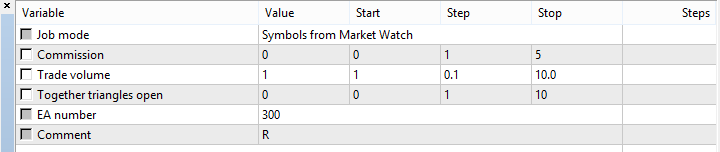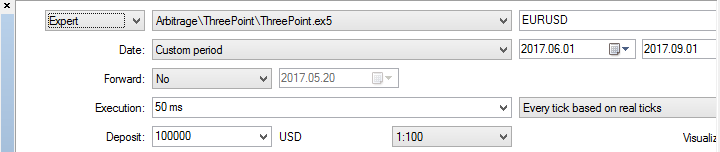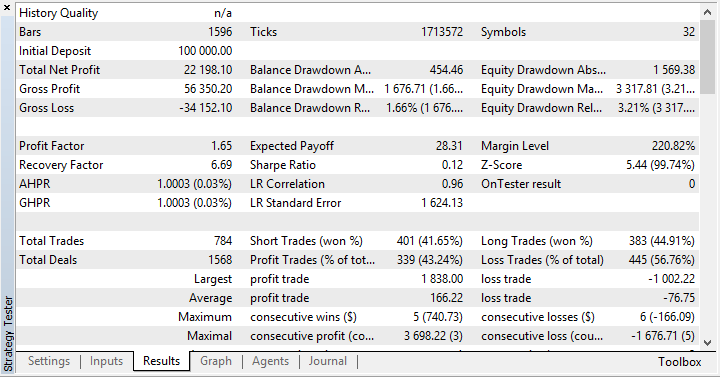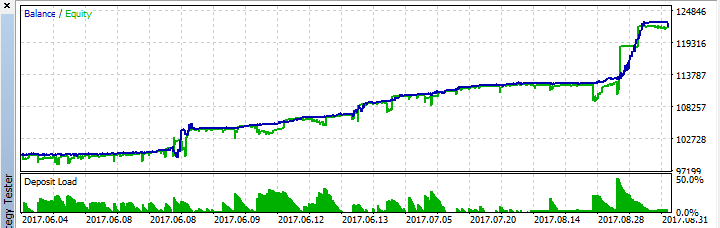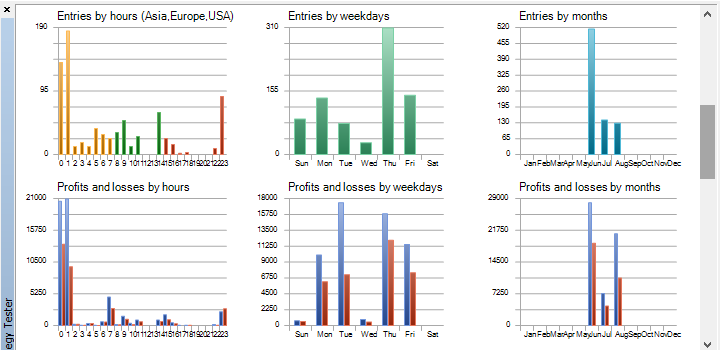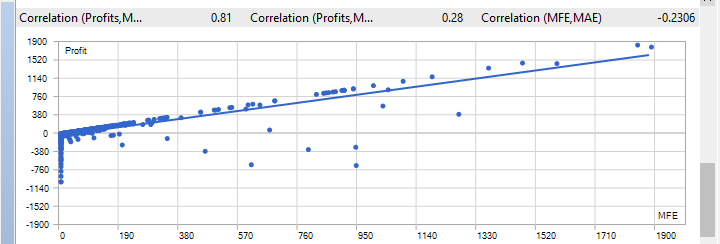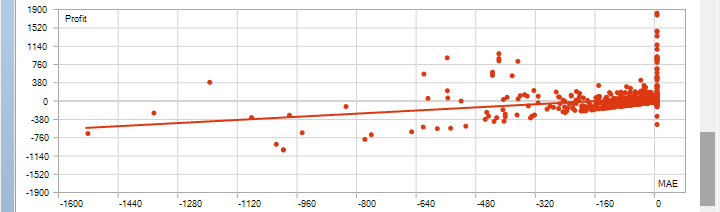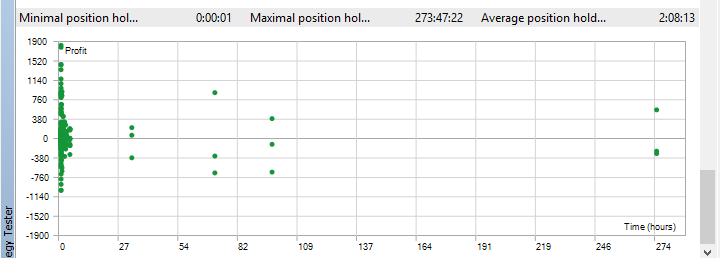It is possible to pass the test in ticks simulation mode and compare with testing on real ticks. We can go even further by comparing test results on real ticks with live action and conclude that the multi-tester is far from reality yet.

The results show that you can rely on 3-4 trades a week on average. Most often, a position is opened at night and the triangle usually features a low-liquidity currency like TRY, NOK, SEK, etc. The robot's profit depends on a traded volume. Since the trades are infrequent, the EA can easily handle large volumes working in parallel with other robots.

The robot's risk is easy to calculate: 3 spreads * number of open triangles.

To prepare the currency pairs we can work with, I recommend first displaying all symbols and then hiding the ones with disabled trading and the ones that are not currency pairs. This can be done faster using the script that is indispensable for fans of multi-currency strategies: https://www.mql5.com/en/market/product/25256

I should also remind you that the history in the tester is not uploaded from the broker's server - it should be uploaded into the client terminal in advance. Therefore, this should be done either independently before testing or using the above script again.

### Development prospects

Can we improve the results? Yes, of course. To do this, we need to make our liquidity aggregator. The drawback of this approach is the necessity to open accounts at multiple brokers.

We can also speed up the test results. This can be done in two ways which can be combined. The first step is to introduce a discrete calculation constantly tracking the triangles, at which the entry probability is very high. The second way is to use OpenCL, which is very reasonable for this robot.

### Files used in the article

# File name Description
1 var.mqh Describing all applied variables, defines and inputs.
2 fnWarning.mqh Checking initial conditions for the EA's correct operation: inputs, environment, settings.
3 fnSetThree.mqh Forming currency pair triangles. The source of the pairs can also be selected here — Market Watch or a file prepared in advance.
4 fnSmbCheck.mqh The function of checking a symbol for availability and other limitations. NB: Trade and quote sessions are not checked in the robot.
5 fnChangeThree.mqh Changing the location of currency pairs in the triangle to form them in a unified way.
7 fnCalcDelta.mqh Considering all separations in the triangle, as well as all additional costs: spread, commissions, slippage.
8 fnMagicGet.mqh Searching for a magic number that can be used for the current triangle.
fnOpenCheck.mqh Checking if the triangle is opened successfully.
10 fnCalcPL.mqh  Calculating triangle profit/loss.
12  fnControlFile.mqh The function maintaining a log file. It contains all openings and closings with necessary data.
13  fnCloseThree.mqh Closing a triangle.
14  fnCloseCheck.mqh Checking if a triangle has closed completely.
15  fnCmnt.mqh Displaying comments on the screen.
16  fnRestart.mqh Checking if there are previously opened triangles when launching the robot. If yes, resuming tracking them.
17  fnOpen.mqh Opening a triangle.
18 Support.mqh Additional support class. It has only one function — counting decimal places for a fractional number.

Translated from Russian by MetaQuotes Software Corp.
Original article: https://www.mql5.com/ru/articles/3150

Attached files |
MQL5.zip (231.93 KB)
Last comments | Go to discussion (28)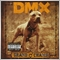| 17 Jun 2018 at 19:43
<Deleted>| 18 Jun 2018 at 13:35
<Deleted>| 18 Jul 2019 at 21:32
hellow sir @Alexey Oreshkin can you please give me your ATR Level indicator source code?| 2 May 2020 at 17:03

Hi i try to use it for over than 1 week and realy try hours. i wanna use it for future contracts F_EURUSD1220, F_EURTRY1220, F_USDTRY1220. It's too much arbitrage price. how can i do? with which doc?

Thank You.| 29 May 2020 at 17:32
I tried this expert on a demo account for tow month and its awesome but it sometimes opens a wrong  positions (open just one position not three positions) and that lead to loss near to 20% of the account balance , dose anyone have a solutions for this error?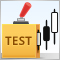Mini Market Emulator or Manual Strategy Tester

Mini Market Emulator is an indicator designed for partial emulation of work in the terminal. Presumably, it can be used to test "manual" strategies of market analysis and trading.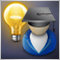Fuzzy Logic in trading strategies

The article considers an example of applying the fuzzy logic to build a simple trading system, using the Fuzzy library. Variants for improving the system by combining fuzzy logic, genetic algorithms and neural networks are proposed.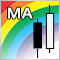Comparing different types of moving averages in trading

This article deals with seven types of moving averages (MA) and a trading strategy to work with them. We also test and compare various MAs at a single trading strategy and evaluate the efficiency of each moving average compared to others.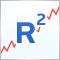R-squared as an estimation of quality of the strategy balance curve

This article describes the construction of the custom optimization criterion R-squared. This criterion can be used to estimate the quality of a strategy's balance curve and to select the most smoothly growing and stable strategies. The work discusses the principles of its construction and statistical methods used in estimation of properties and quality of this metric.